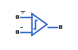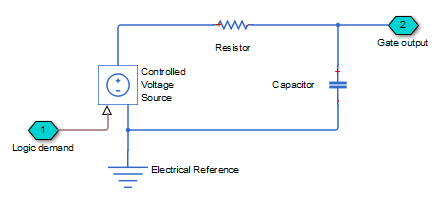# Comparator

Behavioral model of a comparator integrated circuit

•Libraries:
Simscape / Electrical / Integrated Circuits

## Description

The Comparator block is an abstracted behavioral model of a comparator integrated circuit. It does not model an internal transistor-level implementation. Therefore, the block runs quickly during simulation but retains the correct I/O behavior. The block models differential inputs electrically as having infinite resistance and a finite or zero capacitance.

The block models the gate output as a voltage source driving a series resistor and a capacitor that connects to ground. The output pin connects to the resistor-capacitor connection node. If the difference in the inputs is greater than the input threshold voltage, then the output is equal to the High level output voltage (${V}_{OH}$). Otherwise, the output is equal to the Low level output voltage (${V}_{OL}$).The output model is shown in the following illustration.## Assumptions and Limitations

Modeling of the output as a controlled voltage source is representative of a totem-pole or push-pull output stage. To model a device with an open-collector:

1. Connect the output pin to the base of an NPN Bipolar Transistor or PNP Bipolar Transistor block.

2. Set the Output resistance parameter to a suitable value.

## Ports

### Conserving

expand all

Electrical conserving port associated with the comparator non-inverting input.

Electrical conserving port associated with the comparator inverting input.

Electrical conserving port associated with the comparator output

## Parameters

expand all

### Input

The voltage which the difference in the input voltages must be greater than so that the comparator gives a logic output 1.

You can usually find this capacitance value on a manufacturer datasheet. Setting this value to zero can result in faster simulation times.

### Output

The steady-state output voltage, ${V}_{OL}$, when the voltage difference across the inputs is less than or equal to the threshold voltage, and the output current is zero.

The steady-state output voltage, ${V}_{OH}$, when the voltage difference across the inputs is greater than the threshold voltage, and the output current is zero.

This parameter is the ratio of output voltage drop to output current. Set this parameter to $\left({V}_{OH}-{V}_{OH}{}_{1}\right)/{I}_{OH}{}_{1}$, where ${V}_{OH}{}_{1}$ is the reduced output high voltage when the output current is ${I}_{OH}{}_{1}$.

Set this value based on the high-to-low and low-to-high propagation delays.

## Version History

Introduced in R2009b